Deutsche VersionElectric Current, Electric Power, Electrical Voltage

Electricity and Electric Charge

The most common general formulas used in electrical engineering

Basic Formulas and Calculations

Relationship of the physical and electrical quantities (parameters)
Electric voltage V, amperage I, resistivity R, impedance Z, wattage and power P
Volt V, ampere A, resistance and impedance ohm Ω, and watt W

 The nominal impedance Z = 4, 8, and 16 ohms (loudspeakers) is often assumed as resistance R. Ohm's law equation (formula): V = I × R and the power law equation (formula): P = I × V. P = power, I or J = Latin: influare, international ampere, or intensity and R = resistance. V = voltage, electric potential difference Δ V or E = electromotive force (emf = voltage).

 Enter any two known values and press "calculate" to solve for the two others. Please, enter only two values.

 Voltage or volts E or V volts V Amperage or current I amperes, amps A Resistivity or resistance R ohms Ω Wattage or power P watts W For R take impedance Z
 Basic Principles of Electric Circuits Physics Formulary and Electricity Equations Formula wheel ▼ Important formulas Electrical engineering laws Electronic engineering laws Electrical formulas as circle diagram (pie chart)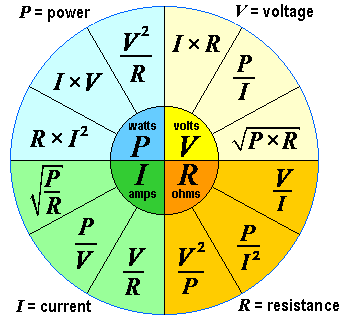The Formula Wheel of Electrical Engineering

 V comes from "voltage" and E from "electromotive force (emf)". E means also energy, so we choose V. Energy = voltage × charge. E = V × Q. Some like better to stick to E instead to V, so do it. For R take Z.

 The 12 most important Formulas: Voltage V = I × R = P / I = √(P × R) in volts V          Current I = V / R = P / V = √(P / R) in amperes A Resistance R = V / I = P / I2 = V2 / P in ohms Ω     Power P = V × I = R × I2 = V2 / R in watts W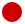The Big Power Formulas            Electrical and mechanical power calculation (strength)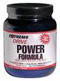Power Formula 1 – Electrical power equation: Power P = I × V = R × I2 = V2 ⁄ R  where power P is in watts, voltage V is in volts and current I is in amperes (DC).  If there is AC, look also at the power factor PF = cos φ and φ = power factor angle  (phase angle) between voltage and amperage.  Electric Energy is E = P × t − measured in watt-hours, or also in kWh. 1J = 1N×m = 1W×s    Power Formula 2 – Mechanical power equation: Power P = E ⁄ t where power P is in watts,  Power P = work / time (W ⁄ t). Energy E is in joules, and time t is in seconds. 1 W = 1 J/s.  Power = force multiplied by displacement divided by time P = F × s / t or  Power = force multiplied by speed (velocity) P = F × v.    Undistorted powerful sound is not found in these formulas. Please, mind your ears!  The eardrum and microphone diaphragms are really only moved by the waves of the  sound pressure. That does not do either the intensity, nor the power or the energy.  If you are in the audio recording business, it is wise not to care much about the energy,  power and intensity as the cause, care more about the effect of sound pressure p  and sound pressure level at the ears and at the microphones and look at the corresponding  audio voltage V ~ p; see: Sound pressure and Sound power − Effect and Cause  Very loud sounding speakers will have a lot of power, but better look closer at the very  important efficiency of loudspeakers. This includes the typical question:  How many decibels (dB) are actually twice or three times as loud?  There is really no RMS power. The words "RMS power" are not correct. There is a calculation  of power which is the multiplication of a RMS voltage and a RMS current.  Watts RMS is meaningless. In fact, we use that term as an extreme shorthand for power in  watts calculated from measuring the RMS voltage. Please, read here:  Why there is no such thing as 'RMS watts' or 'watts RMS' and never has been.  "RMS" power is a rather silly term which has gathered currency among audio people.  Power is the amount of energy that is converted to a unit of time. Expect to pay more when  demanding higher power.André-Marie Ampère was a French physicist and mathematician. The SI unit of measurement of electric current, the ampere, was named after him. Alessandro Giuseppe Antonio Anastasio Volta was an Italian physicist. The SI unit of measurement of electric voltage, the volt, was named after him. Georg Simon Ohm was a German physicist and mathematician. The SI unit of measurement of electric resistance, the ohm, was named after him. James Watt was a Scottish inventor and mechanical engineer. The SI unit of measurement of electric wattage (power), the watt, was named after him.

 In sound engineering there is no Impedance matching or Power matching. In audio we use only high Impedance bridging or Voltage bridging.

 Power is like all energy sizes primarily a calculated value.The word "power amplifier" is a misnomer – especially in audio engineering. Voltage and current can be amplified. The strange term "power amplifier" has become understood to mean an amplifier that is intended to drive a load such as a loudspeaker. We call the product of current gain and voltage gain "power amplification".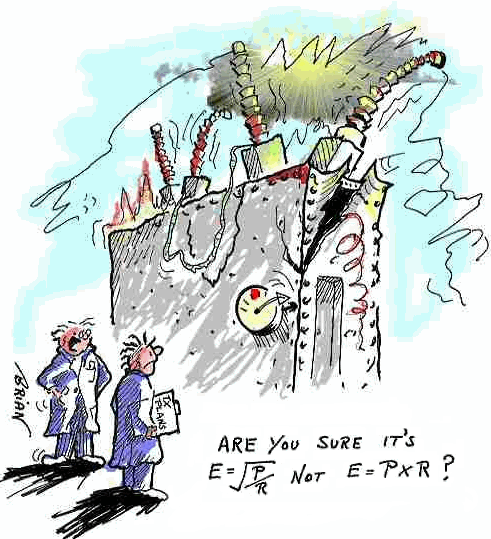Tip: The electric voltage triangle V = I × R (Ohm's law VIR)
Please enter two values, the third value will be calculated.
 Electric voltage V volts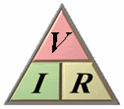Amperage I amps Resistance R ohms V = I × R              I = V / R              R = V / I
The electric power triangle P = I × V (Power law PIV)
Please enter two values, the third value will be calculated.
 Electric Power P watts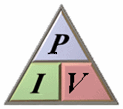Amperage I amps Voltage V volts P = I × V              I = P / V              V = P / I

A magic triangle can be used to calculate all formulas easily. You hide with
a finger the value to be calculated. The other two values show then how to do the calculation.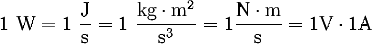ALTERNATING  CURRENT (AC) ~

Vl = line voltage (volts), Vp = phase voltage (volts), Il = line current (amps), Ip = phase current (amps)
Z = impedance (ohms), P = power (watts), φ = power factor angle, VAR = volt-amperes (reactive)

 Current (single phase): I = P / Vp×cos φ Current (3 phases): I = P / √3 Vl×cos φ or I = P / 3 Vp×cos φ Power (single phase): P = Vp×Ip×cos φ Power (3 phases): P = √3 Vl×Il×cos φ or P = √3 Vp×Ip×cos φ
Power factor PF = cos φ = R/(R2 + X2)1/2, φ = power factor angle. For the purely resistive circuit, PF = 1 (perfect).
The apparent power S is calculated according to Pythagoras, the active power P and reactive power Q. S = √(P2 + Q2)

 DC power formulas Voltage V in (V) calculation from current I in (A) and resistance R in (Ω): V(V) = I(A) × R(Ω) The power P in (W) calculation from voltage V in (V) and current I in (A): P(W) = V(V) × I(A) = V2(V) / R(Ω) = I2(A) × R(Ω)   AC power formulas The voltage V in volts (V) is equal to the current I in amps (A) times the impedance Z in ohms (Ω): V(V) = I(A) × Z((Ω) = (| I | × | Z |) and (θI + θZ) The apparent power S in volt-amps (VA) is equal to the voltage V in volts (V) times the current I in amps (A): S(VA) = V(V) × I(A) = (| V | × | I |) and (θV − θI) The real power P in watts (W) is equal to the voltage V in volts (V) times current I in amps (A) times the power factor (cos φ): P(W) = V(V) × I(A) × cos φ The reactive power Q in volt-amps reactive (VAR) is equal to the voltage V in volts (V) times the current I in amps (A) time the sine of the complex power phase angle (φ): Q(VAR) = V(V) × I(A) × sin φ The power factor (FP) is equal to the absolute value of the cosine of the complex power phase angle (φ): PF = | cos φ |

The true power factor and not the conventional 50/60 Hz displacement power factorElectrical Measurement Definitions Quantity Name Definition frequency f hertz (Hz) 1/s force F newton (N) kg·m/s² pressure p pascal (Pa) = N/m² kg/m·s² energy E work joule (J) = N·m kg·m²/s² power P watt (W) = J/s kg·m²/s³ electric charge Q coulomb (C) = A·s A·s voltage V volt (V)= W/A kg·m²/A·s³ current I ampere (A) = Q/s A capacitance C farad (F) = C/V = A·s/V = s/Ω A²·s4/kg·m² inductance L henry (H) = Wb/A = V·s/A kg·m²/A²·s² resistance R ohm (Ω) = V/A kg·m²A²·s³ conductance G siemens (S) = A/V A²·s³/kg·m² magnetic flux Φ weber (Wb) = V·s kg·m²/A·s² flux density B tesla (T) = Wb/m² = V·s/m² kg/A·s²

 The flow of electric charge Q is referred to as an electric current I. The amount of charge per unit time is the change in electric current. A current flows at a constant value I. during the time t, it transports the charge Q = I × t. For a temporally constant power, the relationship between the charge and current: I = Q / t or Q = I × t. Through this relationship, the basic units of amps and second the Coulomb in International System of Units is set. The Coulomb unit can be represented as 1 C = 1 A × s. Charge Q, (unit in ampere-hours Ah), discharge current I, (unit in amperes A), time t, (unit in hours h).

In acoustics we have an "Acoustic equivalent for ohm's law"

Relationships of acoustic sizes associated with plane progressive sound waves

Conversions of many units, like power and energy

[top of page]